# Properties of Matrix Addition and Scalar Multiplication | Class 12 Maths

• Last Updated : 09 Nov, 2020

A matrix is simply a rectangular array or set of elements. The Matrix can be defined as an m*n element in the form of m horizontal lines (rows), n vertical lines (columns) known as the m*n order matrix. Elements can be real, complex, or unknown numbers. An m*n matrix clearly looks like: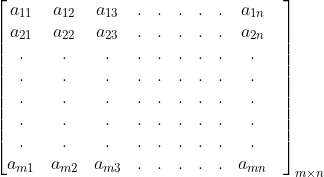In the above figure, a matrix of order m*n is drawn where I and j represent the element’s exact position (i,j).

## Types of Matrices

There are many types of matrices available, a few of them are mentioned below.

### Row Matrix

A matrix having only one row is called a row matrix.

Example:### Column Matrix

A matrix having only one column is known as a column matrix.

Example:### Zero or null Matrix

A matrix having all elements as 0 is known as a zero or null matrix.

Example :### Square Matrix

A matrix having the same no of columns and rows is known as a square matrix.

Example :### Diagonal Matrix

A matrix in which all elements are zero except the diagonal elements is known as a diagonal matrix.

Example :### Scalar Matrix

A special kind of diagonal matrix in which all diagonal elements are the same is known as a scalar matrix.

Example :### Identity Matrix

Identity matrix is a scalar matrix in which all diagonal elements are 1.

Example :## Properties of Zero Matrix

When we add or subtract the 0 matrix of order m*n from any other matrix, it returns the same Matrix. In simple words, “A+0 = A” and “A – 0 = A.”

Example :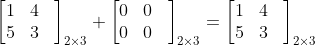Similarly, you can see that the subtraction of a Null matrix from any other matrix will give the other matrix itself as result.

Among all types of matrices, only Zero Matrix rank is always zero in all cases of multiplication.

## Properties of Matrix Addition

A matrix can be added with another matrix if and only if the order of matrices is the same. The addition will take place between the elements of the matrices. The resultant matrix will also be of the same order. That is [A]m×n + [B]m×n = [C]m×n

For example: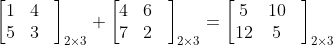There are various unique properties of matrix addition. We will be discussing the below-mentioned properties:

1. Commutative property of addition i.e, A + B = B+ A
2. Associative Property of addition i.e, A+ (B + C) = (A + B) + C
3. Additive identity property. For any matrix A, there is a unique matrix O such that, A+O = A
4. Additive inverse property. When we add a unique matrix –A to A, we get O matrix, A+ (-A) = O.
5. Closure Property of addition A + B = C, where C is a matrix of the same dimensions as A and B.

A, B, and C are Matrix of the same order m*n. To add two Matrices having the same order, simply add the corresponding element of each Matrix. Let’s discuss the addition property of Matrix in detail.

### Commutative Property of Matrix Addition

This property informs that any two matrices of the same order can be added in any way. Suppose there are two matrices A and B of the same order m*n, then the commutative property of matrix addition states that: A + B = B + A

Example :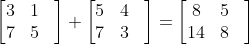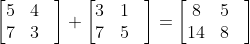From the above example, you can see that Matrix addition follows commutative law.

### Associative Property of Matrix Addition

Similarly, If three matrices have the same order then their position does not matter in addition. Suppose there are three matrices A, B, and C of order m*n, then the associative property of matrix addition states that: A + (B + C) = (A + B) + C

Example: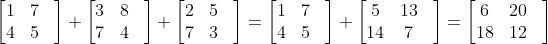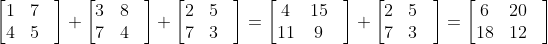From the above example, you can see that matrix addition follows associative law.

### Additive Identity Property of Matrix Addition

We have discussed zero Matrix that O matrix can be added to any matrix for the same result. According to the additive identity property of matrix addition, for a given matrix A of order m*n, there exists an m*n matrix O such that: A + O = A

Here, O is the m*n order zero Matrix.So, if you add a matrix to a zero matrix, then you get the original Matrix.

### Additive Inverse Property of Matrix Addition

There is a rule in Matrix that the inverse of any matrix A is –A of the same order. In simple words, for a given matrix A of order m*n, there exists a unique matrix B such that: A + B = O

Note: This matrix B is equal to –A i.e. B = -A

Therefore, A + (-A) = O

Example: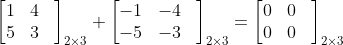We have discussed the various property of the matrix addition. Now we will be discussing some unique properties of matrix scalar multiplication.

## Properties of Matrix Scalar Multiplication

The term scalar multiplication refers to the product of a matrix and a real number. Each entry is multiplied by a given scalar in scalar multiplication. A scalar is a real number in scalar multiplication.

Example: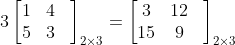Hence, it is clear that Matrix can be multiplied by any scalar quantities.

Matrices multiplication hold some unique properties; a few of them are listed below:

1. Associative Property of Multiplication i.e, (cd)A = c(dA)
2. Distributive Property i.e, c[A + B] = c[A] + c[B]
3. Multiplicative Identity Property i.e, 1.A = A
4. Multiplicative Property of Zero i.e, 0.A = 0 c.0 = 0
5. Closure Property of Multiplication cA is Matrix of the same dimension as A

Note: A is a matrix of order m*n, c, and d are scalars, and O is a zero matrix.

### Associative Property of Matrix Scalar Multiplication:

According to the associative property of multiplication, if a matrix is multiplied by two scalars, scalars can be multiplied together first, then the result can be multiplied to the Matrix or Matrix can be multiplied to one scalar first then resulting Matrix by the other scalar, i.e. (cd)A = c(dA)

For example:

Here we are taking two scalars as 2 and 3.

So,From the above example, you can see that the result is the same in both cases.

### Distributive Property of Matrix Scalar Multiplication

The distributive property clearly proves that a scalar quantity can be distributed over a matrix addition or a Matrix distributed over a scalar addition.

1. c(A + B) = cA + cB

For example:2. (c + d)A = cA + dA### Multiplicative Identity Property of Matrix Scalar Multiplication

If any matrix A is multiplied by the scalar 1, the result is simply the original matrix A.

1.A = ANote: Scalar 1 will be multiplicative identity in scalar multiplication.

### Multiplicative Property of Zero

According to the Multiplicative Property of zero, if any m*n order matrix A is multiplied by scalar 0, then the result is m*n zero Matrix O. This follows the multiplicative properties of zero in the real number system. If any real number x is multiplied by 0, the result is always 0. If any scalar is multiplied to the Zero matrix, the result is the same as the zero Matrix.

0.A = 0c.0 = 0### Closure Property of Matrix Scalar Multiplication

Closure property simply states that if you have a scalar quantity X and a matrix A of the same order m*n, then each element will be multiplied by X.This property states that if any matrix A of order m*n is multiplied by any scalar, then the order of Matrix remains same as m*n.

My Personal Notes arrow_drop_up